# How to Combine Two Columns in Google Sheets

There are often situations when you need to combine data. There can be various reasons why you need to combine data from two columns into one. It can be a requirement for a formula, you just simply want the combination data in two columns. Learning to do it is as simple as following a few steps. It is important to know these formula tips and tricks so that you don’t end up wasting your time as and when required. Continue reading this article as it going to be a perfect guide for you to get started with learning about how to combine two columns in Google Sheets and also about methods to combine cells in Google Sheets.## How to Combine Two Columns in Google Sheets

Keep reading further to find the steps explaining how to combine two columns in Google Sheets in detail with useful illustrations for better understanding.

### What are the Formulas to Combine Two Columns in Google Sheets?

Listed below are the formulas that can be used to combine two columns in a Google Sheets vertically as well as horizontally.

A. Formulas to Combine Horizontal Columns

• =ARRAYFORMULA(A2:A11&”-“&B2:B11)
• =ARRAYFORMULA(CONCAT(A2:A11,B2:B11))

B. Formulas to Combine Vertical Columns

• =FILTER({A2:A11;B2:B11},LEN({A2:A11;B2:B11}))
• ={A2:11;B2:B11}
• =UNIQUE({A2:11;B2:B11}

Further in the article, you will learn how to combine two columns in Google Sheets using these formulas and also some methods that will teach you to combine cells in Google Sheets.

Also Read: Difference Between Rows and Columns in Excel

### How to Combine Two Columns in Google Sheets?

Let’s begin with the methods to combine two columns vertically on Google Sheets:

#### Method 1: Use ARRAY FORMULA

This method can be used when you have data in two columns that are supposed to be combined with a space, comma (,), or a hyphen (-) in between. For instance, have a look at the data sample given below.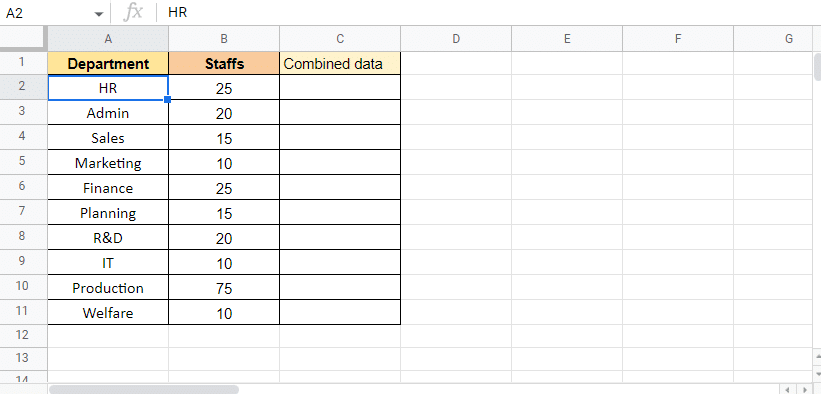Here, we have a list of departments and staff of the respective departments. We will be using the ARRAY formula to combine both data columns using a hyphen (-) in between. Below mentioned are the steps to get started with the process:

1. Start to apply the formula by typing equal to (=)ARRAY in cell C2 as that is where we want the target value.2. Select the Department column and add an ampersand (&) and a separator (-) to select the next column.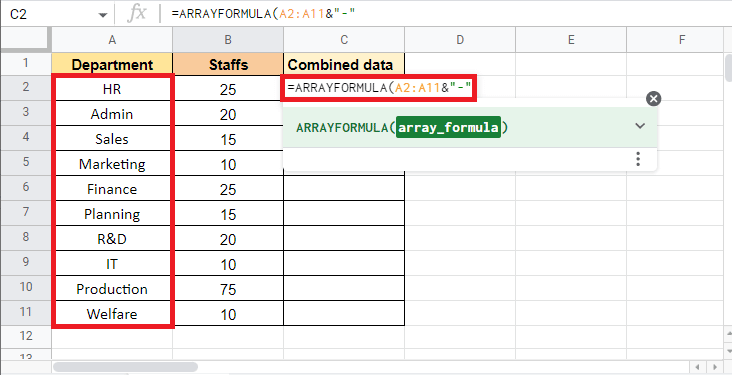3. Add an ampersand (&) and select the Staffs column.4. Close the bracket to complete the ARRAY Formula.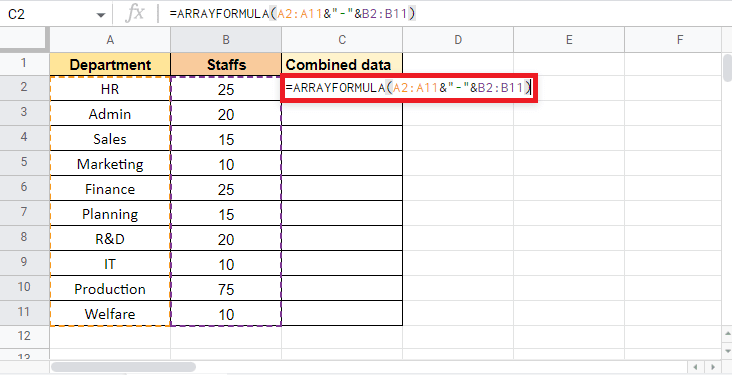5. Press the Enter key to get the result.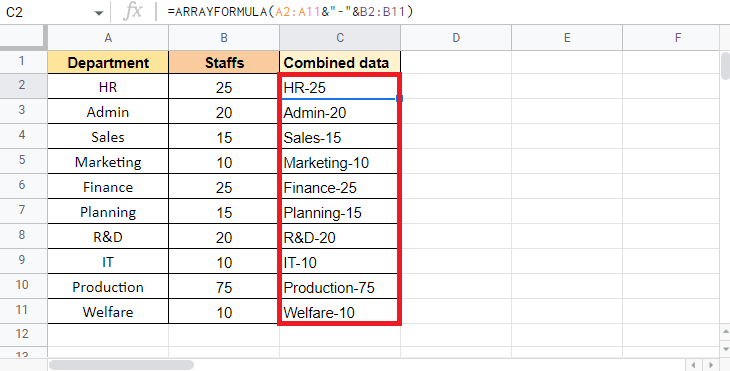Note: Here we have used a hyphen (-) as a separator you can use a comma (,), space ( ), or vertical bar (|) accordingly.

This is how you can use the ARRAY FORMULA to combine two columns into one. Further in this article, you will also read about how to combine multiple cells into one column

#### Method 2: Use ARRAY FORMULA with CONCAT Function

ARRAY FORMULA with CONCAT functions is one of the other ways in which you can use to combine two columns. The only limitation of this method is that you cannot combine the columns with a separator. However, this method will be useful when the combination of cells does not require a separator. In this case, we will be using the below-mentioned data that contains Department Code and ID No.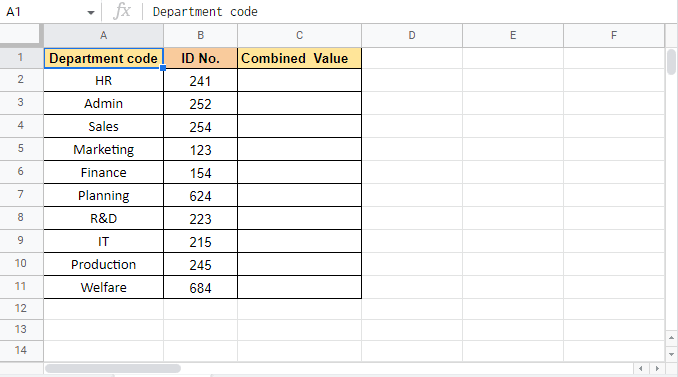Let’s proceed with the steps of this method:

1. Start by applying the ARRAY FORMULA by typing equal to (=) ARRAY in cell C2 where we want the target value.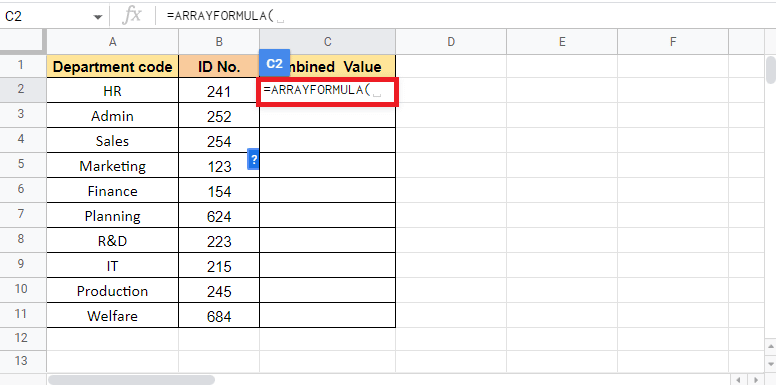2. Now, type CONCAT to get started with the CONCAT function.3. To enter value1, select the Department Code column and add a comma (,) to move to the next value.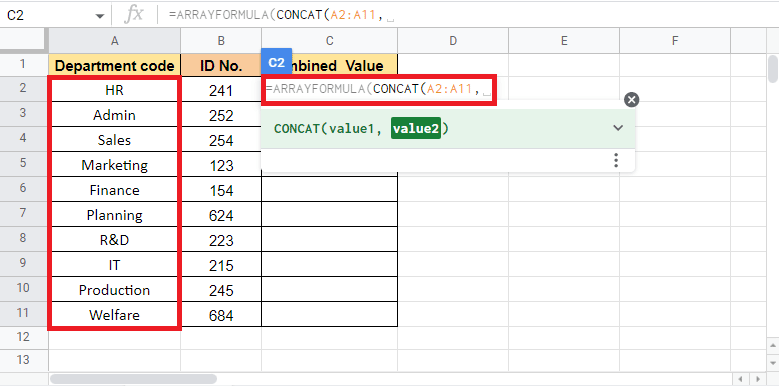4. For value2, select ID No. column and close the bracket to complete the formula.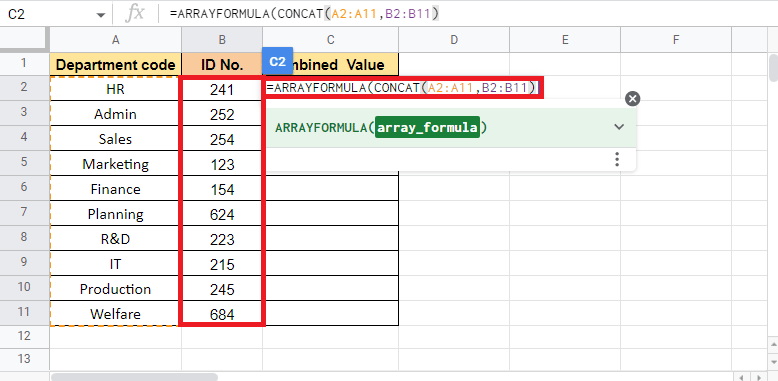5. Press Enter key to get the target value.This is how to combine two columns in Google Sheets using the ARRAY FORMULA with the CONCAT function. Let’s move on to the next method to merge cells in Google Sheets.

#### Method 3: Use FILTER with LEN Function

The FILTER with the LEN function is used in situations when you need to combine one column with another vertically. One advantage of using this method is it eliminates the blank cells within the range. One such example is what we will be looking at here. The below-given data consists of employee names from Shift 1 and Shift 2. By using the FILTER with the LEN function, we will see how to combine multiple cells into one column.Listed below are the steps to use FILTER with the LEN function:

1. Start by entering the =FILTER function in Cell C2 where need the target value.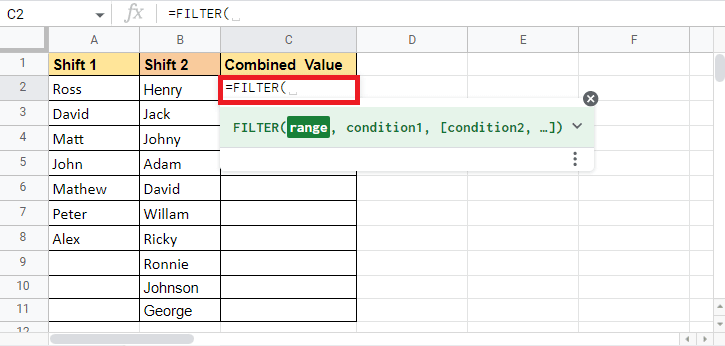2. Open curly brackets and select the Shift 1 column and add a semi-colon (;).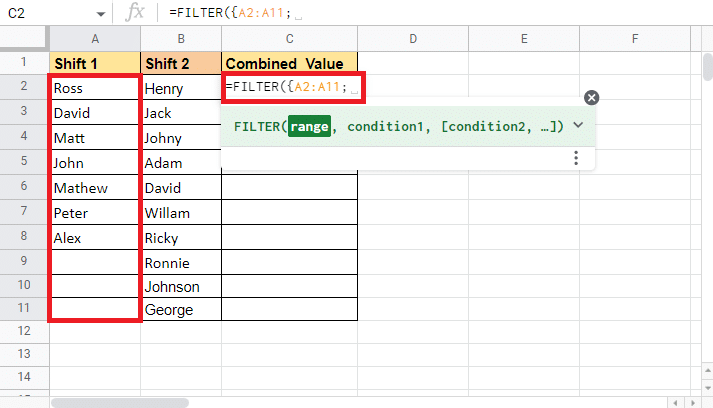3. Select the next column which is the Shift 2 column and close the curly bracket.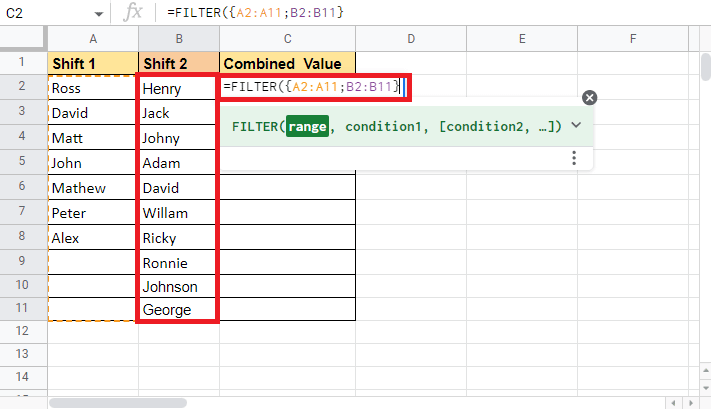4. Add a comma (,) and get started with the LEN function by typing LEN.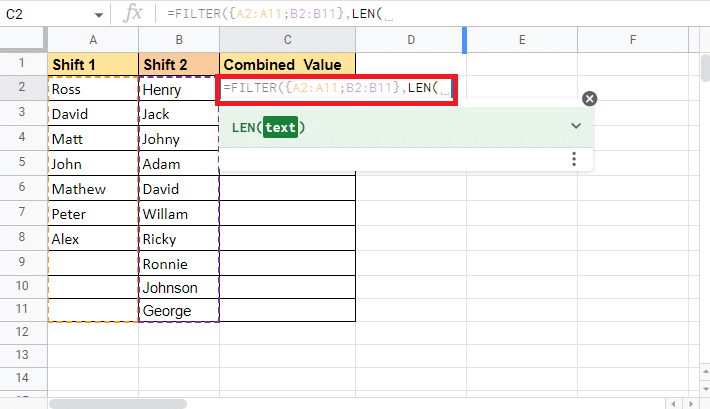5. Open a curly bracket and select shift 1 column again.6. Add a semi-colon (;) and select the shift 2 column again.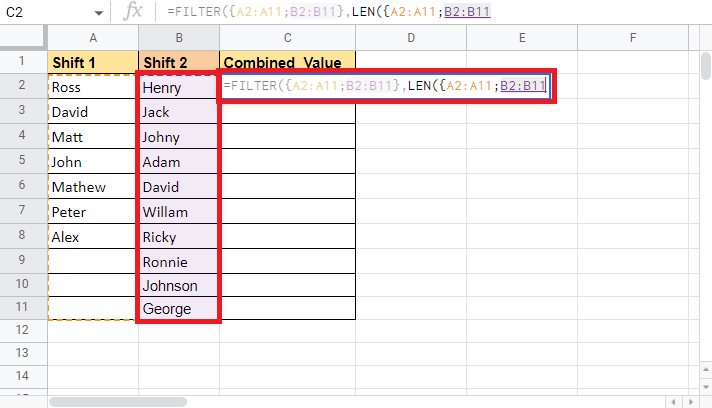7. Close the curly bracket and the bracket of the LEN formula to complete it.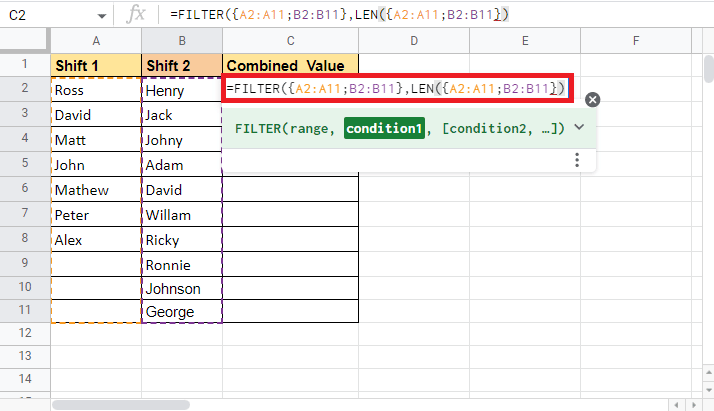8. Close the bracket for the Filter function.9. Press Enter key to see the target values in place.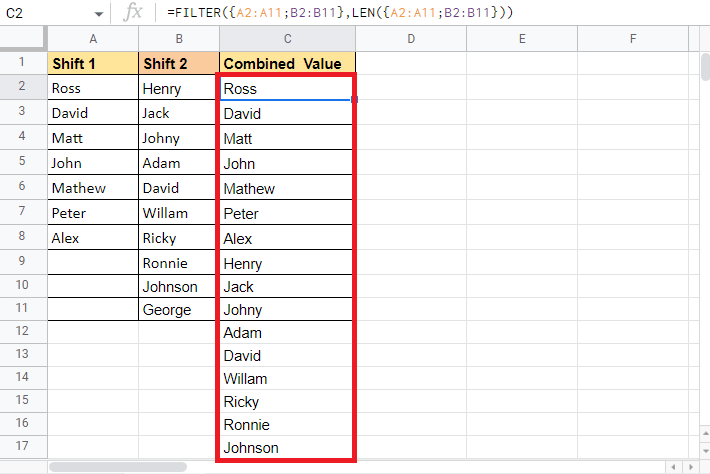This is how you can use the FILTER function with the LEN function to vertically combine cells in Google Sheets. Now we have a look at the next method to see how to combine multiple cells into one column.

Also Read: How to Freeze Rows and Columns in Excel

#### Method 4: Use Curly Brackets and Semi-colon

Using curly brackets and semi-colon is one of the easiest methods for vertically merging or combing columns into one. This method turns out useful when you do not want to make any changes to the source data. Using curly brackets and semi-colon enables the users to stack up the data as it is. To explain this method the data we will be using is the same one that we used for the above-mentioned method. We just have made some new duplicate entries to see how the formula unfolds. This is the sample data you can see a few duplicate entries.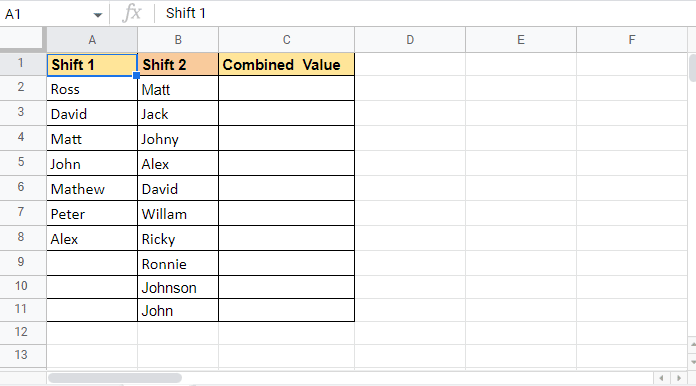Follow the below-mentioned steps to see how this method can be used to merge cells in Google Sheets:

1. Start with an equal to (=) and then open curly brackets in cell C2 where you want the target value.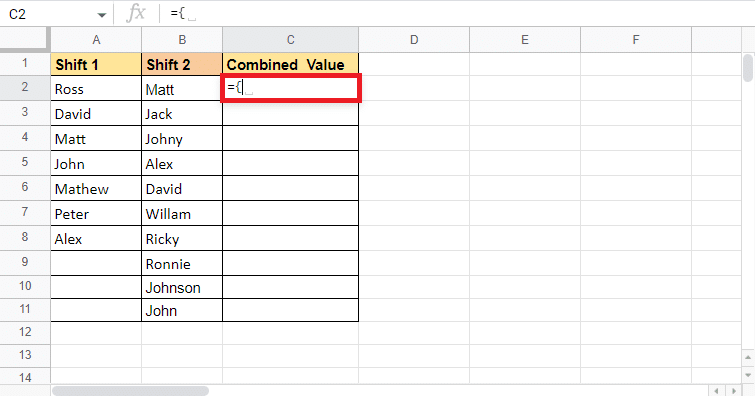2. Select the first column which is the Shift 1 column in the data.3. Add a semi-colon (;) and select the second column which is Shift 2.4. Close the curly bracket.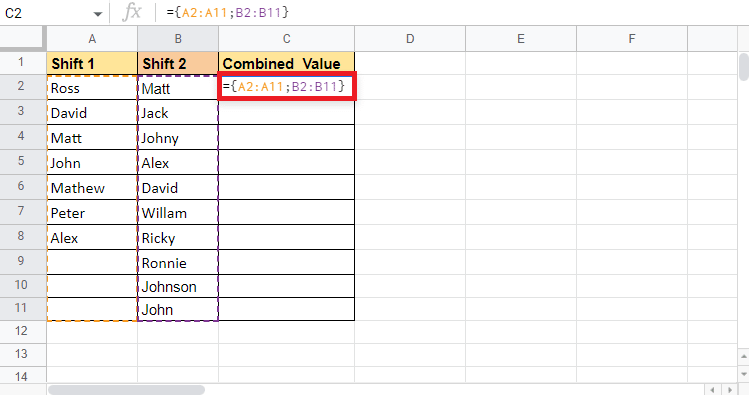5. Press Enter key to get the target value.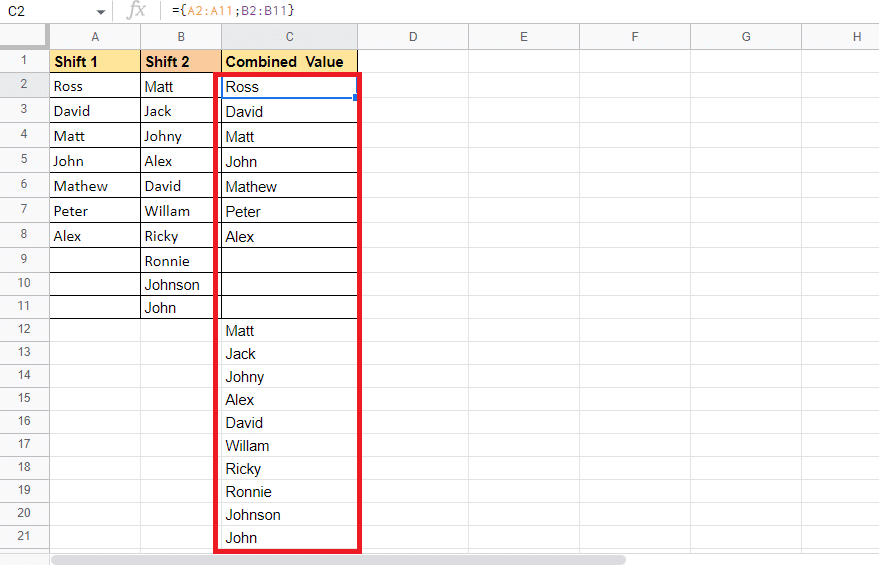This is how you use this method to combine cells in Google Sheets. Though it stacks the data as required it also contains blank cells and duplicate details. If this is not a method that will work for you, you can try the next method.

#### Method 5: Use UNIQUE Function

The UNIQUE function is one of the methods that can help you combine columns vertically. This method is extremely useful when you want to remove duplicate and blank cells, unlike the above-mentioned method that stacks all the data as given. Therefore, we will be using the same data sample as used above. Let’s begin with the steps that can be used to perform this method.

1. Start by putting an equal to (=) and type the UNIQUE formula in cell C2 which is where we want the target value.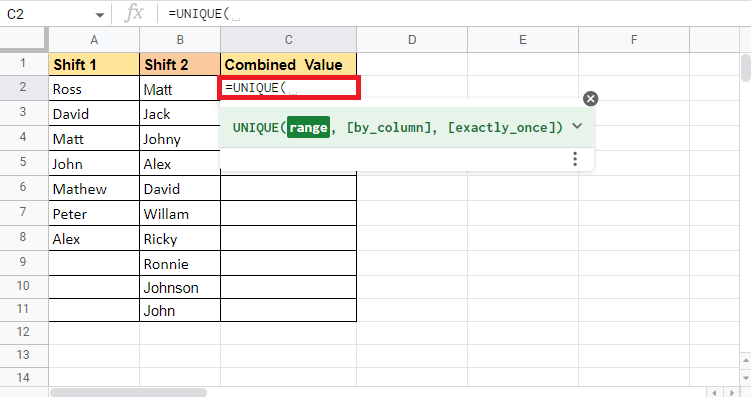2. Open a curly bracket and select the first column Shift 1.3. Add a semi-colon (;) and select the second column Shift 2.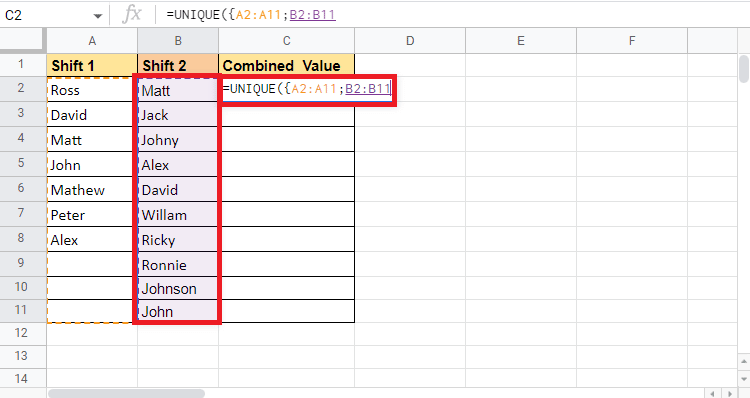4. Complete the formula by adding a curly bracket and a bracket.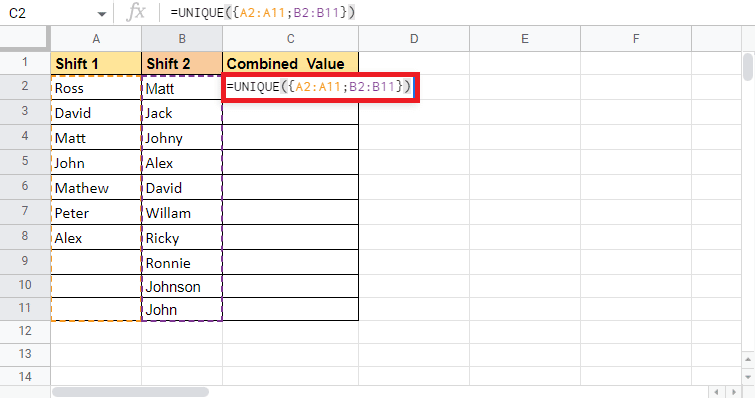5. Press the Enter key to get the target value.As you can see, there is just one entry with one name. This method removes the duplicates and blank cells. There is only one blank cell in the target value that separates the values of different columns. Moving on we will be having a look at the how CONCATENATE function can be used to combine data from two columns into one.

#### Method 6: Use CONCATENATE Function

Using CONCATENATE function is another method that can be used as an alternative to the above-mentioned methods. This method slightly functions in a different manner than the rest. As in the rest of the methods we selected the whole column that we wanted to combine whereas with this function you will combine the cells and copy and paste the formula throughout the cell to combine the columns. Another point to be noted while using this method is that you will have to add an additional column if you require a separator. This separator can be a space ( ), comma (,), hyphen (-), vertical bar (|), etc. The data sample that we are going to use contains the Employee name, Separator, and Incentive. We will be combining these three columns.Let’s proceed with the steps to see how this method functions:

1. Put an equal to (=) and start by typing CONCATENATE in cell D2 where we want the target value.2. Select the first cell that has to be combined. Here, it is A2.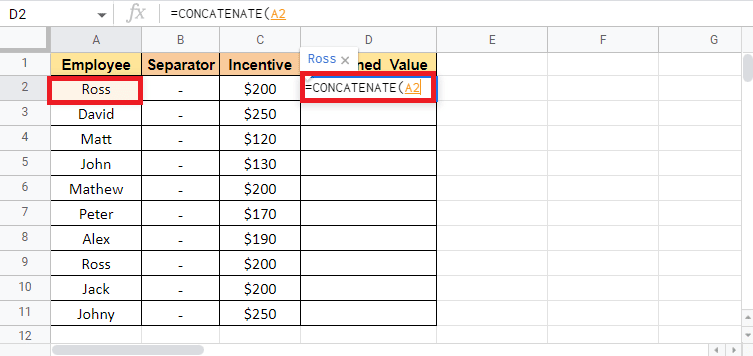3. Add a comma (,) and select the separator cell if you want to add any.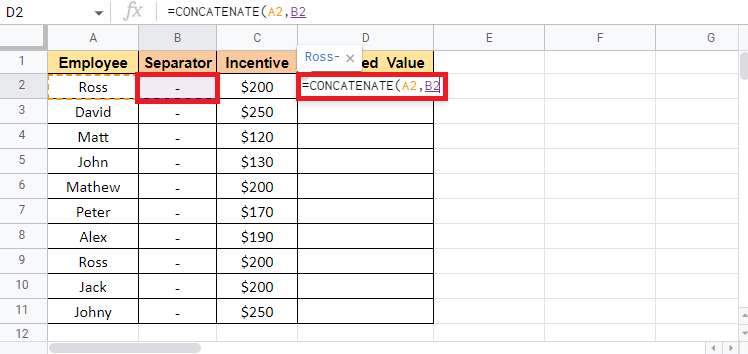4. Add comma (,) and select the third cell that needs to be combined which is cell C2 in this case.5. Close the bracket to complete the formula.6. Press Enter key to see the target value.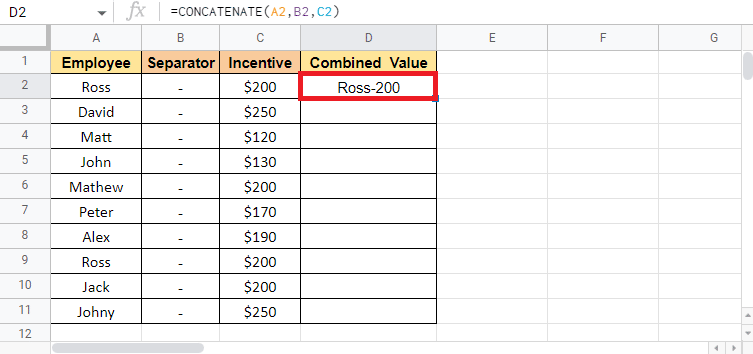7. Drag the corner of the D2 cell to copy the formula throughout the column.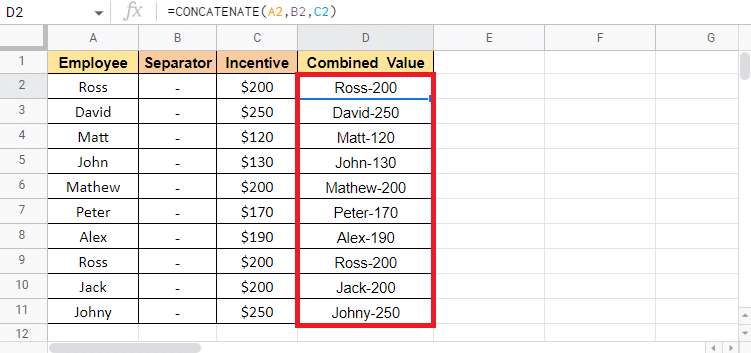Note: If you do not want the separator column in between after combining the value, you can hide the column. If you want to delete the separator column you can cut and paste the Combined Value column. While pasting select the paste value only option. If you do not follow this option you will lose the combined value in case you delete the separator column

These were the methods to combine two or more columns into one vertically and horizontally using the above-mentioned methods.

Also Read: 3 Ways to Combine Multiple PowerPoint Presentation Files

#### Method 7: Use Ampersand with Separator

Using an ampersand with a separator is one of the easiest ways to merge cells in Google Sheets and then combine columns if needed. To explain this method, we will be using the set of data that contains the Employee’s Name and Surname. We will use this method to get the full name in the third column.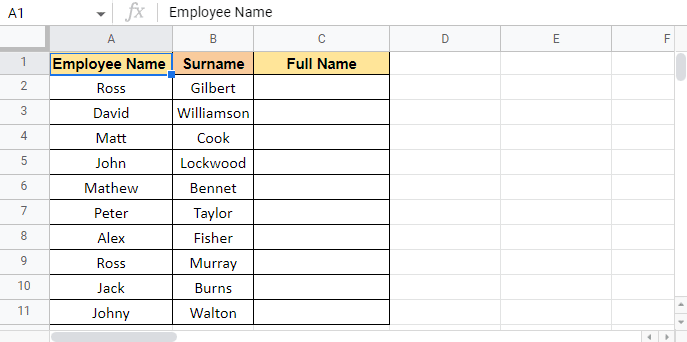Mentioned below are the steps to get started with this method:

1. Put an equal to (=) in the C2 cell where you want the target value.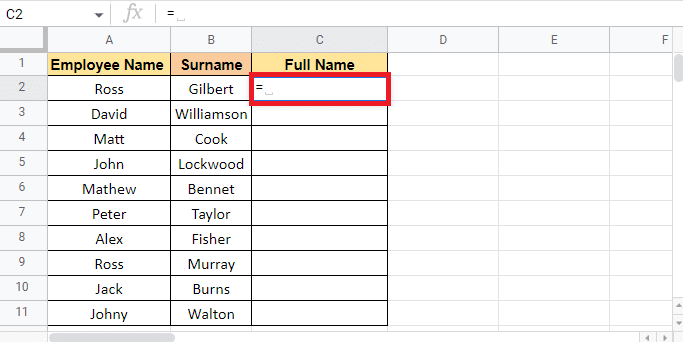2. Select the first cell that has to be combined which is A2, add an ampersand (&), and in quotations add space ( ) as a separator.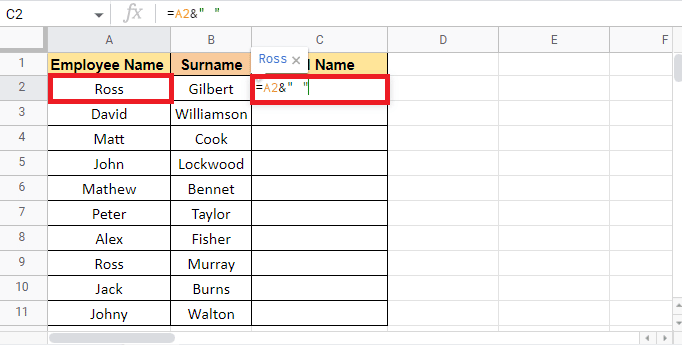3. Put an ampersand (&) again, and select the second cell that has to be combined which is B2.4. Press Enter key to get the full name.5. Apply the formula through the column by dragging the corner of cell C2.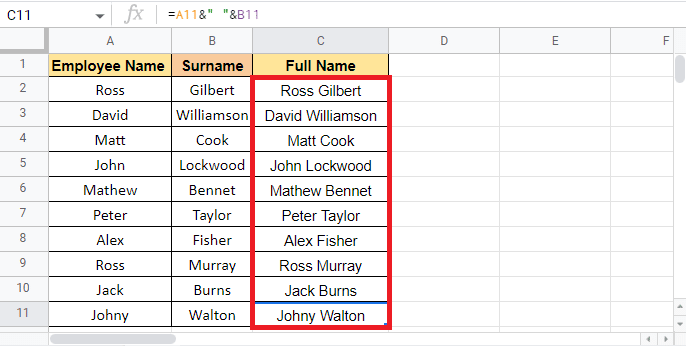Note: Instead of using space ( ) as a separator you can also use other separators accordingly as mentioned above in the article.

Now we will move to the next method that you might also find helpful for combing columns in Google Sheets.

#### Method 8: Use JOIN Function

Using JOIN functions is also as easy as using the above-mentioned methods. Though the syntax of this formula may sound new to you as it contains terms like delimiter which is nothing but the separator. To explain this method we will be using the same data that we used in the above-mentioned method. Let’s proceed with the steps straight away.

1. Put an equal to (=) and start typing the JOIN formula in the cell where you want the target value.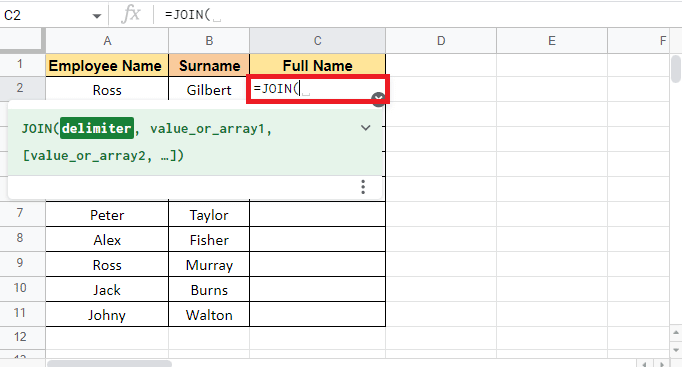2. For the delimiter argument, add space ( ) in quotations and put a comma (,) to move to the next argument.3. Select the first cell A2 that needs to be combined and add a comma (,).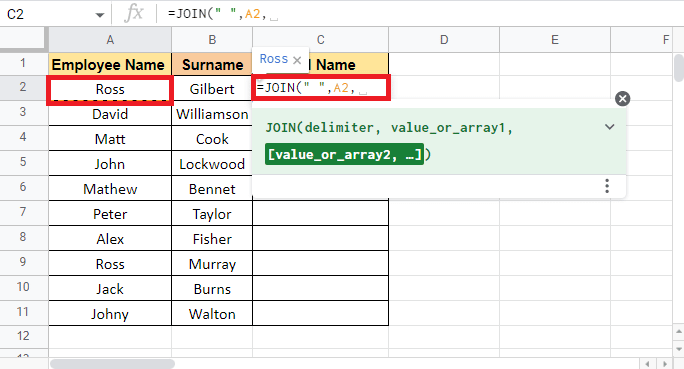4. Select the second cell B2 and close the bracket to complete the formula.5. Press the Enter key to get the target value.6. Apply the formula through the column by dragging the corner of cell C2.Recommended:

This article was all about the methods that you can use to combine multiple cells and columns into one. Each method caters to different data types and can be used accordingly. We hope that this guide was helpful and you were able to learn how to combine two columns in Google Sheets. Let us know which method worked for you best. If you have any queries or suggestions, then feel free to drop them in the comments section below

#### How to Change Your Email on Instagram

Jassweb always keeps its services up-to-date with the latest trends in the market, providing its customers all over the world with high-end and easily extensible internet, intranet, and extranet products.